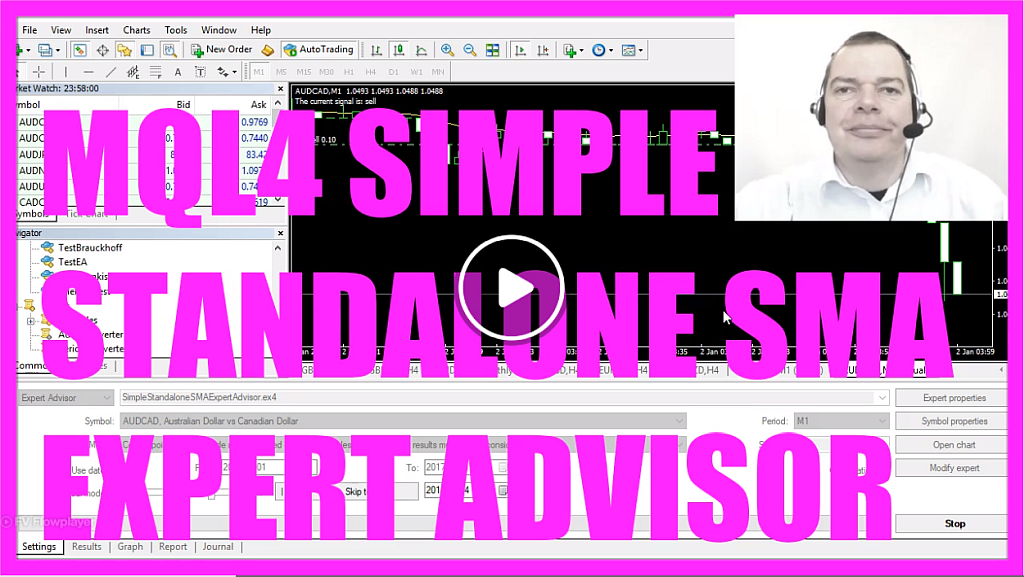In this tutorial, we will discuss a basic Moving Average strategy for Metatrader 4. The strategy will determine whether to open a trade based on the position of the current price relative to the Moving Average.

## Signal Variable Initialization

First, we define a string variable named `signal`. This variable will be used to determine if we have a buy or sell signal.

mql4
```string signal =""; ```

## Calculating the Moving Average

The Moving Average provides an average of prices over a specified number of periods. Here, we calculate the Moving Average for the last 20 candles using the `iMA` function.

mql4
```double MyMovingAverage = iMA(_Symbol, _Period, 20, 0, MODE_SMA, PRICE_CLOSE, 0); ```

## Determining Buy and Sell Signals

We compare the Moving Average to the current price. If the Moving Average is below the current price, it indicates a buy signal. If it’s above, it indicates a sell signal.

mql4
```if (MyMovingAverage<Close) { signal="buy"; } if (MyMovingAverage>Close) { signal="sell"; } ```

## Order Execution

If the signal is “buy” and there are no open orders, a buy order for 10 Microlot is placed. Similarly, if the signal is “sell”, a sell order is placed.

mql4
```if (signal=="buy" && OrdersTotal()==0) OrderSend (_Symbol,OP_BUY,0.10,Ask,3,0,Ask+150*_Point,NULL,0,0,Green); if (signal=="sell" && OrdersTotal()==0) OrderSend (_Symbol,OP_SELL,0.10,Bid,3,0,Bid-150*_Point,NULL,0,0,Red); ```

## Chart Output

Finally, the current signal is displayed on the chart for clarity.

mql4
```Comment ("The current signal is: ",signal); ```

## That is basically it

In this tutorial, we have detailed the creation of a basic Moving Average strategy in MQL4. This strategy uses the Moving Average to determine the position of the current price and decide on trade actions. Testing in a demo environment before live trading is recommended.